# 1 The standard form of a quadratic equation

• Slides: 9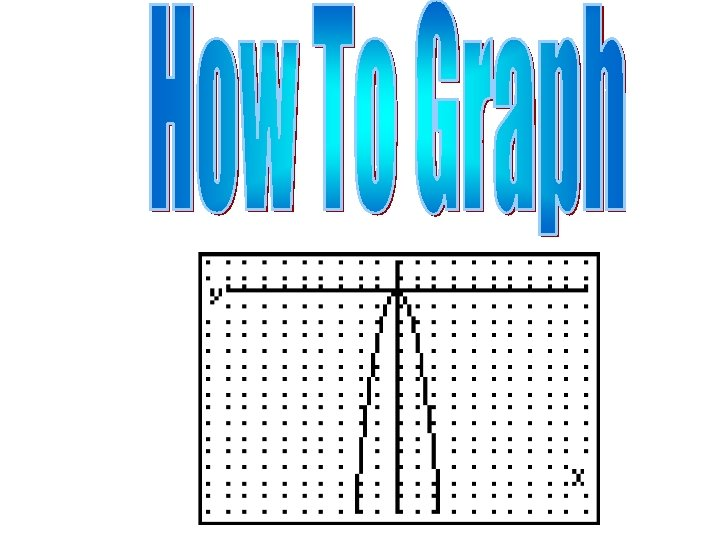1. The standard form of a quadratic equation is y = ax 2 + bx + c. 2. The graph of a quadratic equation is a parabola. 3. When a is positive, the graph opens up. 4. When a is negative, the graph opens down. 5. Every parabola has a vertex. For graphs opening up, the vertex is a minimum (low point). For graphs opening down, the vertex is a maximum (high point). 6. The x-coordinate of the vertex is equal to .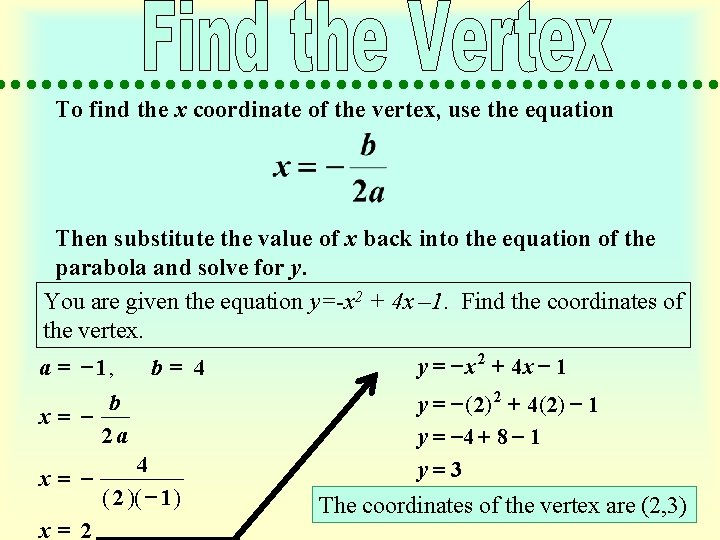To find the x coordinate of the vertex, use the equation Then substitute the value of x back into the equation of the parabola and solve for y. You are given the equation y=-x 2 + 4 x – 1. Find the coordinates of the vertex. y = - x 2 + 4 x - 1 a = - 1, b= 4 b = x 2 a 4 x= ( 2 )( - 1 ) x= 2 y = - ( 2) 2 + 4 ( 2) - 1 y = -4 + 8 - 1 y=3 The coordinates of the vertex are (2, 3)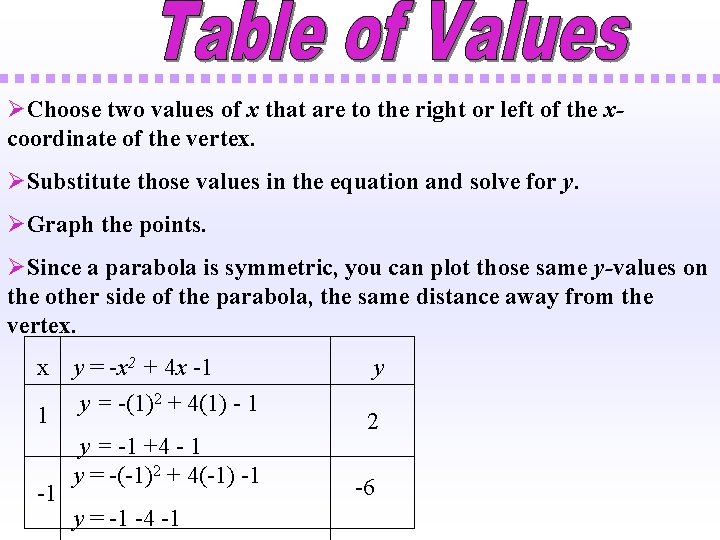ØChoose two values of x that are to the right or left of the xcoordinate of the vertex. ØSubstitute those values in the equation and solve for y. ØGraph the points. ØSince a parabola is symmetric, you can plot those same y-values on the other side of the parabola, the same distance away from the vertex. x y = -x 2 + 4 x -1 2 + 4(1) - 1 y = -(1) 1 -1 y = -1 +4 - 1 y = -(-1)2 + 4(-1) -1 y = -1 -4 -1 y 2 -6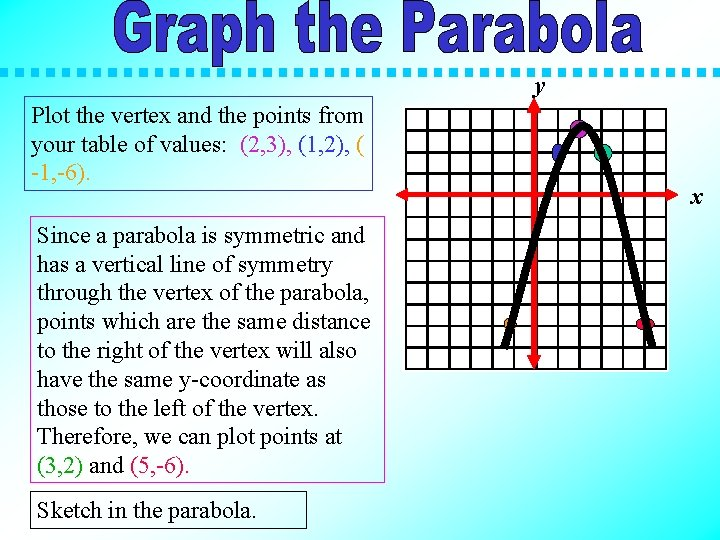y Plot the vertex and the points from your table of values: (2, 3), (1, 2), ( -1, -6). Since a parabola is symmetric and has a vertical line of symmetry through the vertex of the parabola, points which are the same distance to the right of the vertex will also have the same y-coordinate as those to the left of the vertex. Therefore, we can plot points at (3, 2) and (5, -6). Sketch in the parabola. xFind the vertex of the following quadratic equations. Make a table of values and graph the parabola.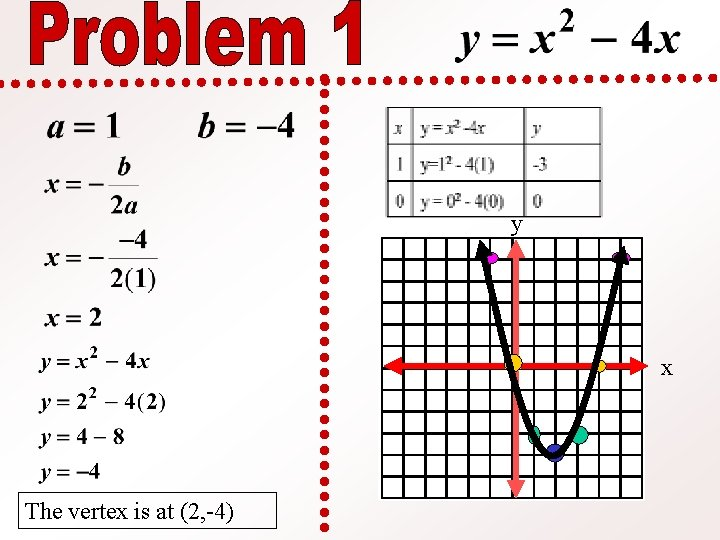y x The vertex is at (2, -4)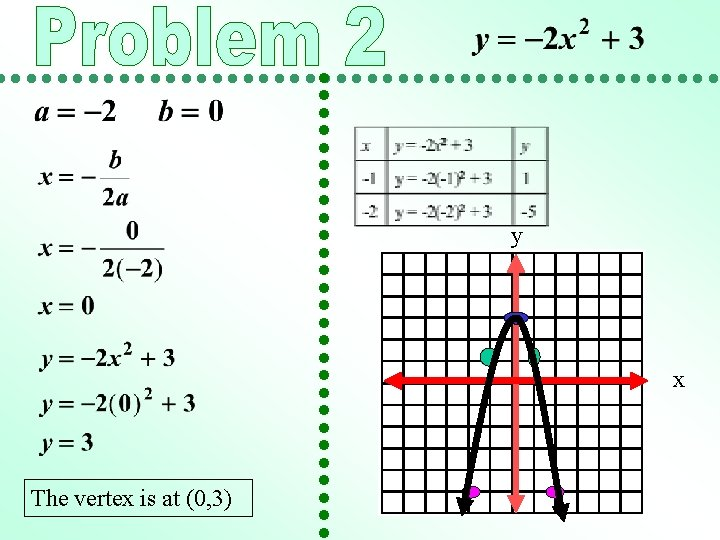y x The vertex is at (0, 3)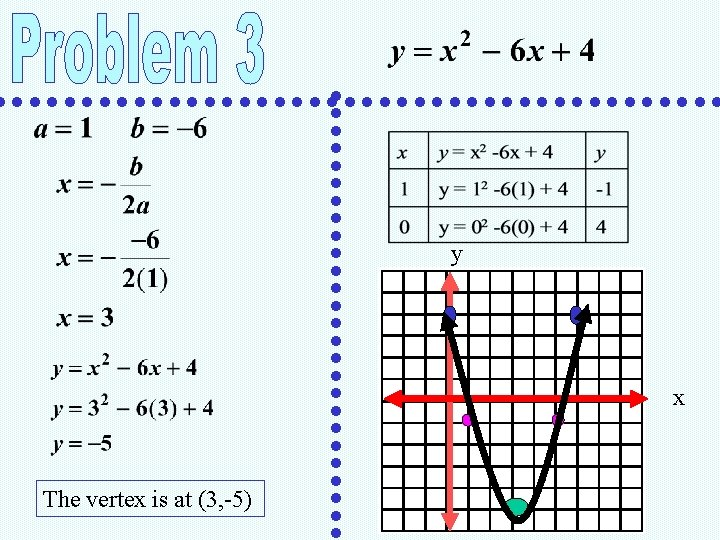y x The vertex is at (3, -5)